# Embrace the ellipsis

In my classes and research group, I work with many novice R users who ask a plethora of great questions. Some of the common questions include things like, “Why do you have to quote a package name when you install it but don’t you have to quote it when you attach (i.e. load) it?” Or, “How do you know which package a function comes from if you don’t use the double colon, i.e., ::?” These sorts of questions indicate that students are comprehending the material, learning the language, and beginning to recognize important patterns.

In my classes, we frequently dissect documentation and comb over function arguments. Students are often curious about how certain arguments will affect the way a function runs, and they routinely vocalize these inquiries. Questions about the ellipsis – the ... used in many R functions – however, almost never come up. It is treated like a mysterious artifact that ought to be avoided.Figure 1: “The Ishumura and the Artifact…must be destroyed

Or perhaps students suspect the power of these dots – much like the Army of the Dead in Lord of the Rings – but seek to avoid unnecessary risks.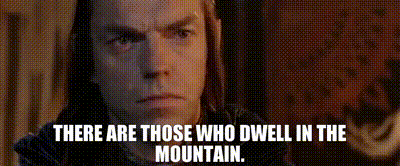Figure 2: Cost benefit analysis

Since I rarely have students utilize arguments which are not explicitly listed by a high level function, maybe they assume it isn’t relevant and is more of a comical catch-all like how “et cetera” is used in movie The King and I: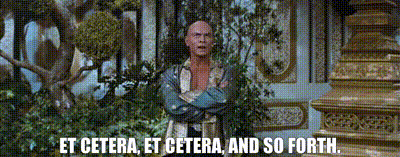Figure 3: I sure do think of this quote (and my mom who says the phrase exactly like the king) every time I see the ellipsis

This is despite the fact that many functions my students see indeed use the ellipsis. To be fair, while I had been aware of the ellipsis and its use for quite a while, I had avoided it much in the way the miners in Dead Space should have avoided the Artifact aside from occasionally passing arguments to lower level functions in ggplot2 or tmap. In a recent project, however, I found the ellipsis – along with extracting and manipulating objects created from it – to have incredible value for one task in particular: constructing helpers and wrappers with sensible defaults around functions like those in ggplot2 which have many optional arguments.

This post demonstrates how the ellipsis, or the “dots” (e.g., ...), can be used in function creation. There is a great introductory post on the ellipsis here, but it lacks tangible examples and memes, so I wrote this post to fill that gap. I contend that the ellipsis is more like Figure 2 than it is like Figure 1 or Figure 3. It is not a portal to a dark universe nor meaningless filler but a convenient way to construct functions which pass user arguments to other functions, particularly when the function creator wants to supply reasonable defaults yet allow these to be changed. Advantageously, one need not be Gondorian royalty to wield it’s power.

## Functions for everyone

For my Quantitative Methods in Geography class, I created a package called haffutils which contains numerous functions intended to lower R’s barrier of entry for beginners. This reduces the amount of code that students have to write and makes data analysis and visualization simpler. One function that students use is designed for creating density plots. Aside from the function I created, there are two simple ways to create a basic density plot; one (a) involves using functions from the built-in base and stats packages:

x <- rnorm(100)

plot(density(x))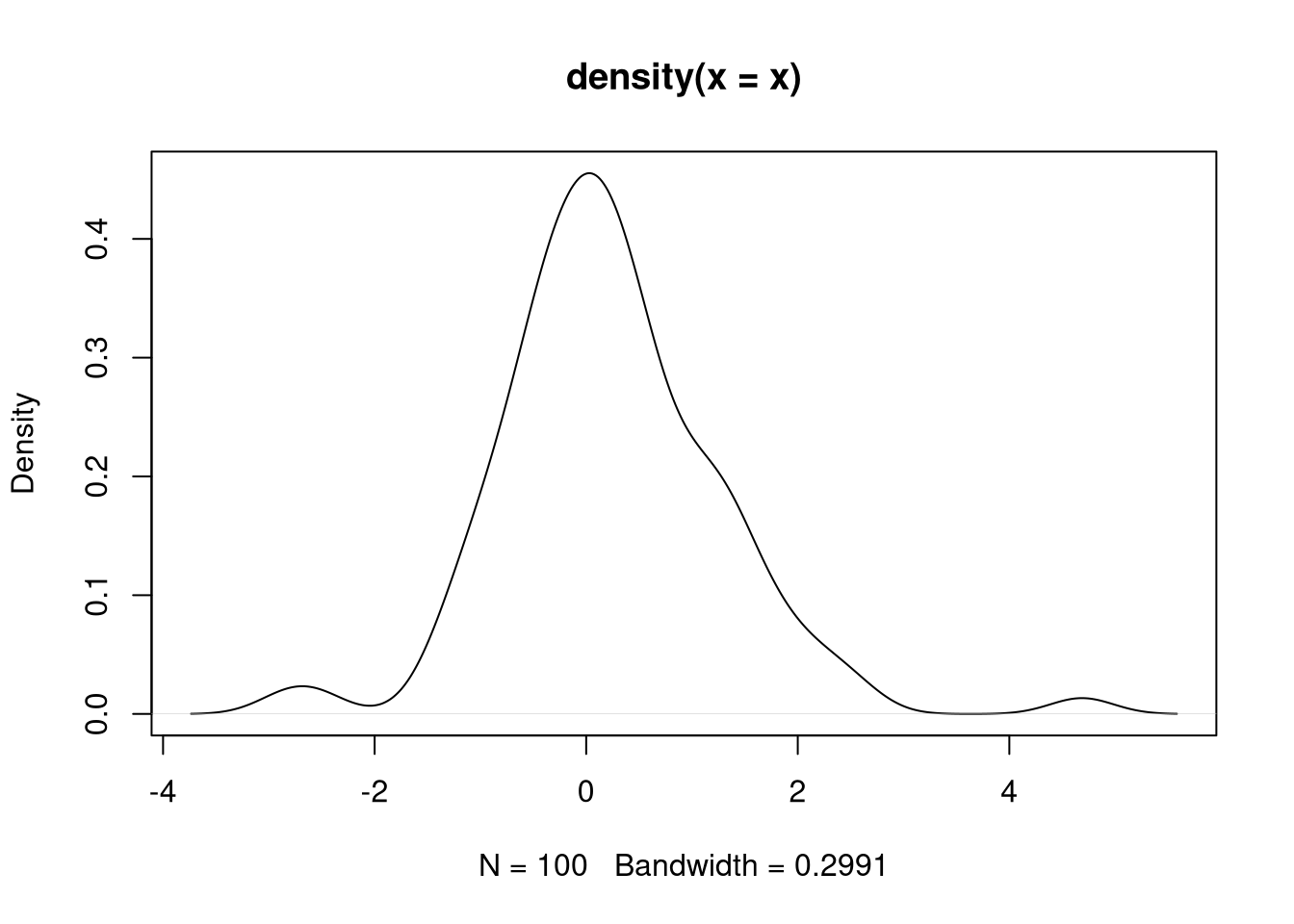Figure 4: Ugly density plot

And (b) another involves using ggplot2:

library(ggplot2)

x <- rnorm(100)

df <- data.frame(x)

ggplot(df, aes(x)) +
geom_density()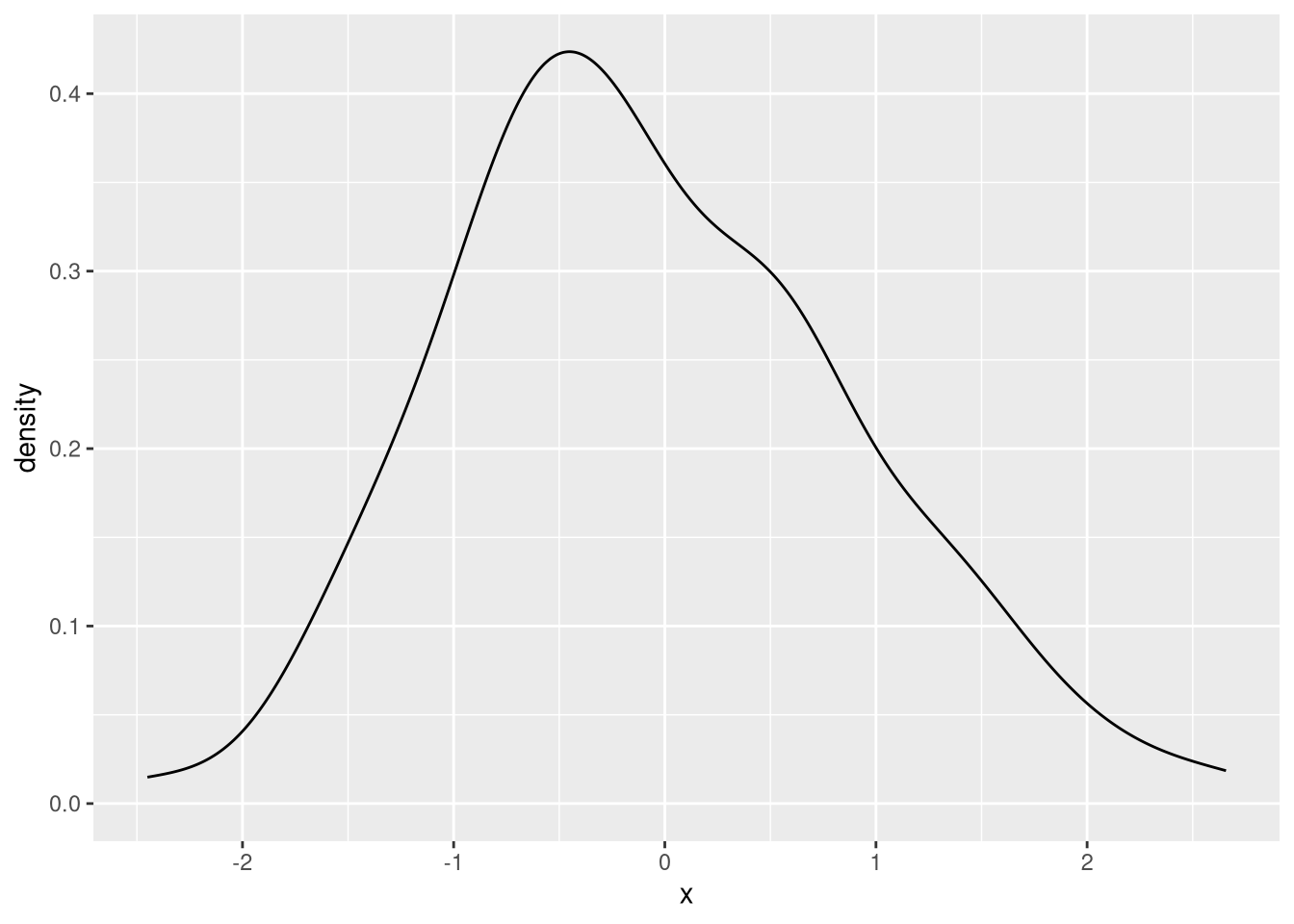Figure 5: Slighly less ugly density plot

The first option is ugly, and unlike using the hist function to create a histogram, it requires a small extra step in computing the density before plotting it. The second option involves way too much technical debt for beginner R users, especially in a class focused on quantitative methods in geography rather than on R itself. While ggplot2’s grammar of graphics is powerful and translatable to many other visualization types, I simply don’t have time to explain to students why a vector must first be converted to a data frame, how the aes function works, et cetera, et cetera, and so forth. Further, to create a minimally nice looking density plot, I think the ggplot example above needs some color and transparency:

library(ggplot2)

x <- rnorm(100)

df <- data.frame(x)

ggplot(df, aes(x)) +
geom_density(fill = "orange", alpha = 0.5)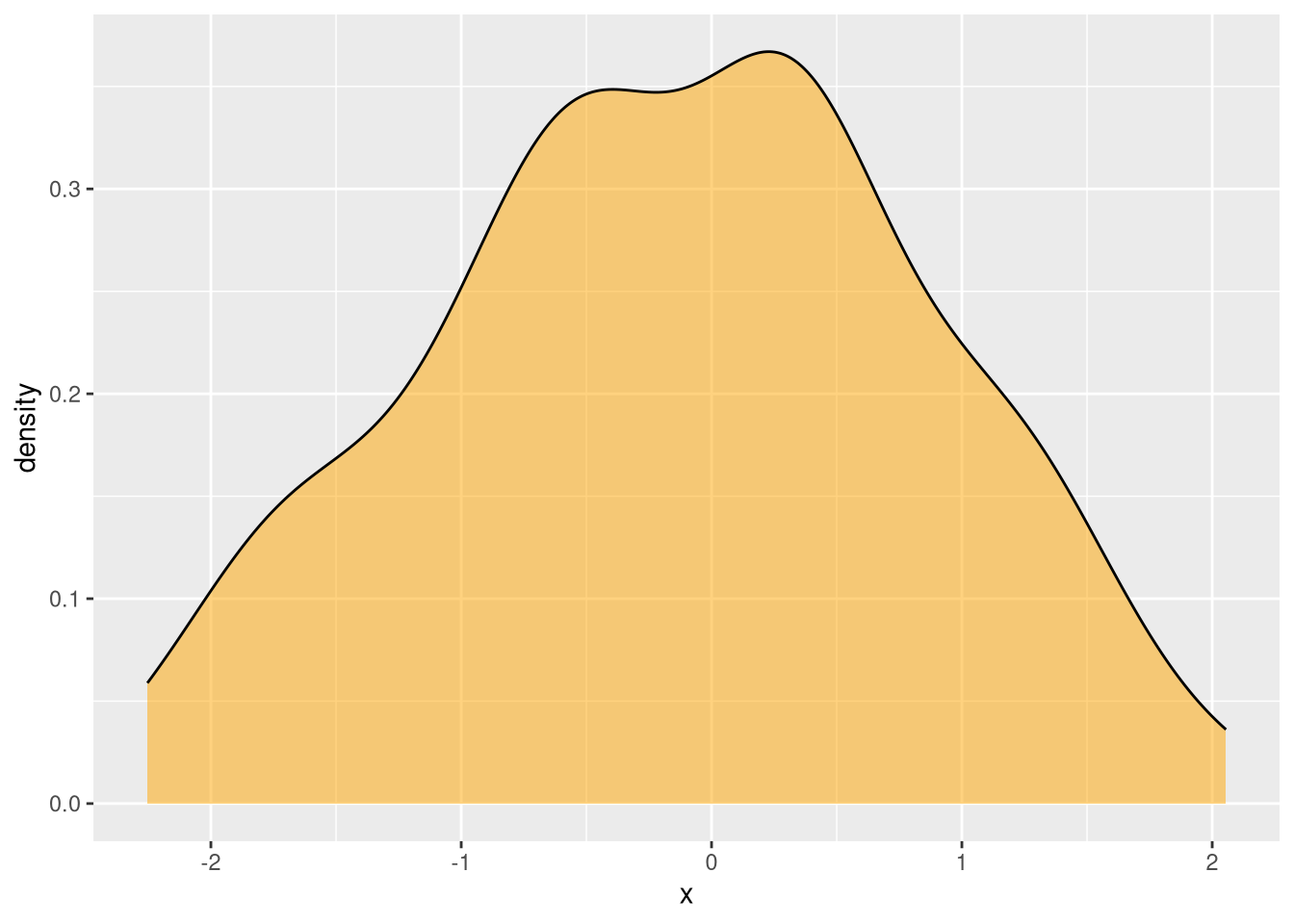Figure 6: A beautiful density plot which is cumbersome to create for novice R users

To resolve this issue, I wanted to create a single function option that would (a) require one only argument, (b) have that required argument be a numeric vector rather than a data frame, and (c) look nice with reasonable visual defaults, particularly with color and transparency. So I created a wrapper around geom_density called pretty_dens (short for “pretty density plot”) which allows for this:

library(haffutils)

x <- rnorm(1000)

pretty_dens(x)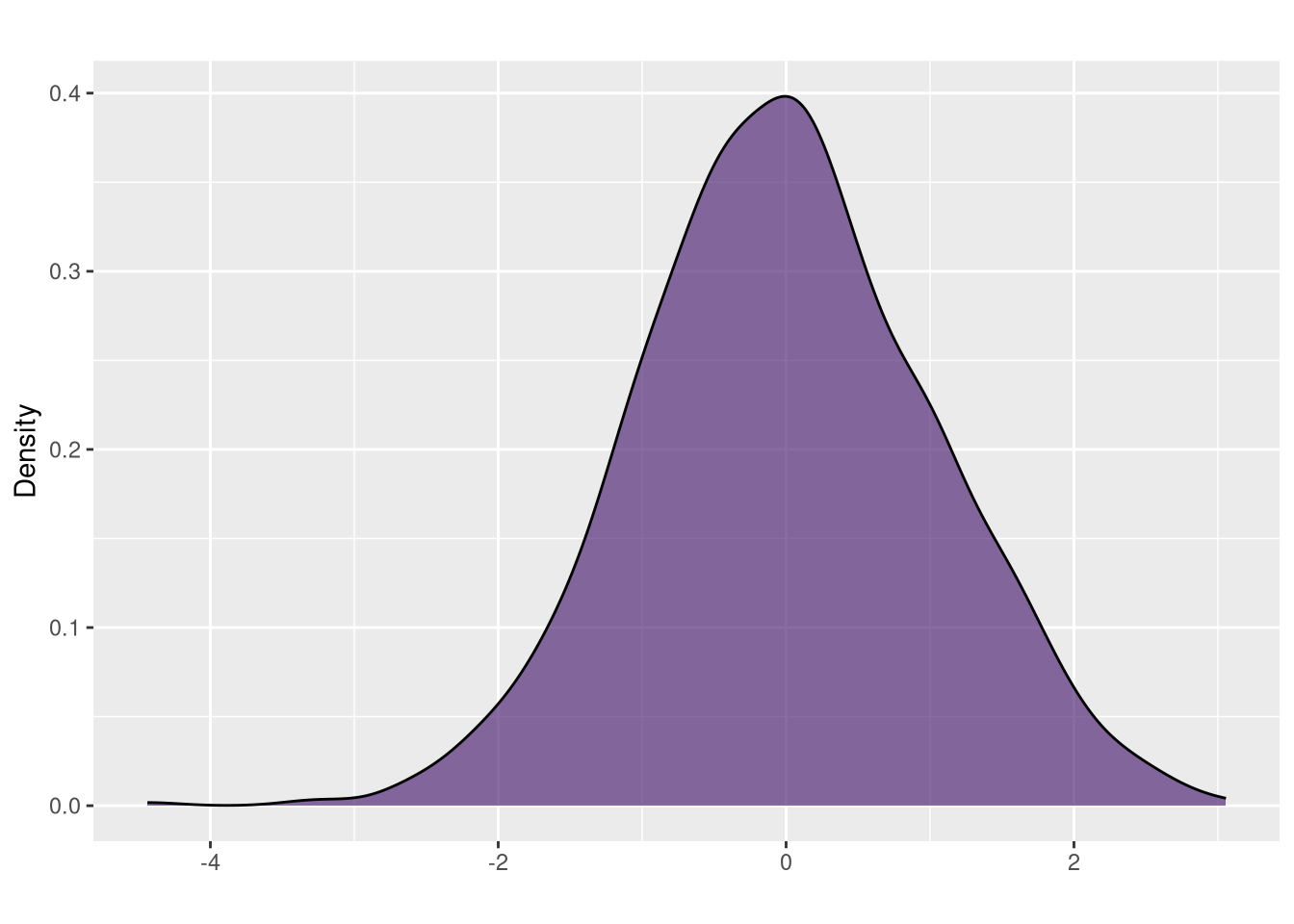Figure 7: A beautiful density plot which is easy to create for novice R users

The color is selected randomly from the viridis palette using this in the body of the pretty_dens function:

fill <- viridis::viridis(100) %>% sample(1)

This makes things fun and interesting since it will produce a different color every time the pretty_dens function is invoked, but written this way, it has a disadvantage in that it prohibits users from selecting their own color. This is where the ellipsis come in handy when creating functions, though leveraging its potential is not intuitive.

Awkwardly, the ellipsis returns some unexpected things when using it in ways that are commonly used to interact with other R objects, namely typing the object name in the console to see its contents or by using the class function to inspect the object’s data type. Consider the example of a relatively basic R object, the open paren (i.e., ():

> (

returns

.Primitive("(")

And

> class(()

returns

 "function"

But trying these operations with the ellipsis returns something different:

> ...

returns

Error: '...' used in an incorrect context

And

> ...
Error: '...' used in an incorrect context

And

> class(...)
Error: '...' used in an incorrect context

This is because the “dots” (as they are called in the documentation) – and ?... does indeed return its documentation – or the “ellipsis” as it is more commonly referred to in the R community, is not a function or variable. The ellipsis is a syntactic element and a reserved word in R.

But the confusion goes even further: suppose you use the ellipsis in function creation to allow for the passing of arguments from your R function to another. E.g.,

#' @usage pretty_dens(x)
#' @param x numeric vector
#' @param ... other arguments passed to geom_density
#' @return a plot
#' @keywords visualization
#' @export
#' @examples
#' pretty_dens(rnorm(1000))

pretty_dens <- function(x, ...) {

ggplot2::ggplot(df, ggplot2::aes(x=x)) +
geom_density(...)
}

Utilization like this:

x <- rchisq(1000, 4)

pretty_dens(x)

Or this:

x <- rchisq(1000, 4)

pretty_dens(x, fill = "cyan")

would work as intended. But if you modify the value of fill in the body of the function it would obviously override anything the user supplies:

#' @usage pretty_dens(x)
#' @param x numeric vector
#' @param ... other arguments passed to geom_density
#' @return a plot
#' @keywords visualization
#' @export
#' @examples
#' pretty_dens(rnorm(1000))

pretty_dens <- function(x, ...) {
fill <- viridis::viridis(100) %>% sample(1)

df <- data.frame(x=x)

ggplot2::ggplot(df, ggplot2::aes(x=x)) +
ggplot2::geom_density(fill=fill, ...)
}

In the past, I’ve been confronted with similar situations where I need to check for the existence of certain user arguments and make adjustments if they’re not present. A great example would be the case where a user has not supplied a color for a plot. This example works but it’s unwieldy:

#' @usage pretty_dens(x)
#' @param x numeric vector
#' @param ... other arguments passed to geom_density
#' @return a plot
#' @keywords visualization
#' @export
#' @examples
#' pretty_dens(rnorm(1000))

pretty_dens <- function(x, fill=viridis::viridis(100) %>% sample(1), ...) {
df <- data.frame(x=x)

ggplot2::ggplot(df, ggplot2::aes(x=x)) +
ggplot2::geom_density(fill=fill, ...)
}

This would get out of hand with any more arguments that have their defaults supplied this way. The code used to create the fill should go in the body of the function rather than in its argument list. But this does not work:

#' @usage pretty_dens(x)
#' @param x numeric vector
#' @param ... other arguments passed to geom_density
#' @return a plot
#' @keywords visualization
#' @export
#' @examples
#' pretty_dens(rnorm(1000))

pretty_dens <- function(x, ...) {
if (!exists("fill")) {
fill <- viridis::viridis(100) %>% sample(1)
}

df <- data.frame(x=x)

ggplot2::ggplot(df, ggplot2::aes(x=x)) +
ggplot2::geom_density(...)
}

Since !exists("fill") will always return TRUE in this case, even if the user supplies a value for fill. The hasArg() function can be used instead to check for user arguments, even those included in the ellipsis.

#' @usage pretty_dens(x)
#' @param x numeric vector
#' @param ... other arguments passed to geom_density
#' @return a plot
#' @keywords visualization
#' @export
#' @examples
#' pretty_dens(rnorm(1000))

pretty_dens <- function(x, ...) {
if (!hasArg("fill")) {
fill <- viridis::viridis(100) %>% sample(1)
}

df <- data.frame(x=x)

ggplot2::ggplot(df, ggplot2::aes(x=x)) +
ggplot2::geom_density(fill=fill, ...)
}

This works fine if the user does not pass an argument for fill; in this case, !hasArg("fill") is TRUE, and fill is assigned to a variable. But what if the user does supply an argument to fill? An error is returned:

object 'fill' not foundFigure 8: Where be the fill?

If you’ve made it this far, you can probably see that fill is “hiding” in the ellipsis – R can tell that it has been passed as an argument through !hasArg("fill"), but because it’s not a required argument, it’s value is encapsulated in ..., not in the variable fill that is being supplied to geom_density. Fortunately, we can look for ellipsis variables by using this:

list(...)

If this is used in the console outside of a function, it will throw an error as demonstrated earlier, but inside the function, it can be used to retrieve ellipsis arguments. As one might expect, list(...) conveniently works when debugging. So, if a breakpoint is set in a function like this (using Emacs/ESS and how it visually shows breakpoints):

pretty_dens <- function(x, ...) {
df <- data.frame(x=x)
B>
ggplot2::ggplot(df, ggplot2::aes(x=x)) +
ggplot2::geom_density(...)

We can inspect user arguments once the function is used. With arguments supplied as:

pretty_dens(rnorm(100), fill="orange")

From the debugger on the console we can use list(...) which will return:

$fill  "orange" This feels like that moment in Ocarina of Time when you obtain the Lens of Truth.Figure 9: Use ess-bp-set and then C-c C-c and then list(...) The trick then becomes modifying the arguments if they are not supplied in a way that allows you to avoid explicitly referencing an object like fill as a standalone variable. So, the recommended way to do this is to put every user-supplied ellipsis argument in a variable: args <- list(...) Then, search the variable args for the existence of those arguments you want to modify, then modify args (which is a list) accordingly. Then, the trick is to use do.call() to apply each argument from args to the appropriate function. In my case, it would look like this: pretty_dens <- function(x, ...) { args <- list(...) if (!"fill" %in% names(args)) { args$fill <- viridis::viridis(100) %>% sample(1)
}

dens <- do.call(ggplot2::geom_density, args)

Then, the density plot, dens, is added to the “chain” of ggplot2 functions:

  df <- data.frame(x=x)

ggplot2::ggplot(df, ggplot2::aes(x=x)) +
dens
}

And it works! This allows the user to specify their own argument for fill that gets passed to geom_density, while supplying a reasonable default if they do not supply one. The whole function has a bit more to it and looks like this:

#' Create a simple, nice looking density plot using a vector as input
#'
#' Base R does not have a one line/one function option for creating density
#' plots. Similar to pretty_hist(), this function takes a vector as input and
#' produces a nice looking density plot of a single variable using ggplot2 under
#' the hood.
#' @usage pretty_dens(x)
#' @param x numeric vector
#' @param title character string, optional title
#' @param xlab character string, optional x-axis label
#' @param ylab character string, optional y-axis label
#' @param ... other arguments passed to geom_density
#' @return a plot
#' @keywords visualization
#' @export
#' @examples
#' pretty_dens(rnorm(1000))
#'
pretty_dens <- function(x, title="", xlab="", ylab="Density", ...) {
## get ellipsis arguments
args <- list(...)

## set some reasonable defaults for geom_histogram (ellipsis arguments)
if (!"fill" %in% names(args)) {
args$fill <- viridis::viridis(100) %>% sample(1) } if (!"alpha" %in% names(args)) { args$alpha <- 0.65
}

dens <- do.call(ggplot2::geom_density, args)

## remove na values
x <- x[!is.na(x)]

## create data frame for ggplot2
df <- data.frame(x=x)

ggplot2::ggplot(df, ggplot2::aes(x=x)) +
dens +
ggplot2::ggtitle(title) +
ggplot2::xlab(xlab) +
ggplot2::ylab(ylab)
}

In addition to supplying a default for fill, I also set the opacity to 65% with the alpha argument. The function also removes NA values. While this is a bit dangerous for sophisticated users, this function is intended to be used for teaching purposes, not in a production environment. I also have a pretty_hist function which creates histograms, and it has some other considerations which don’t apply to density plots (e.g., number of bins). I may add to these functions over time and/or create new functions which leverage list(...) as needs arise.

Aside from its utility, extracting objects from list(...) is fun and gives me an excuse to do some debugging. With this knowledge of the ellipsis in mind, you should now put aside [your fear of the ellipsis]. Become who you were born to be [as an R function creator].Figure 10: You will suffer me!